# Useful Results

In the following,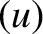represents a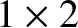vector, whereas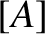represents a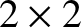matrix. The elements ofandare denoted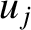and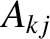, respectively, where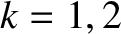indexes the rows, and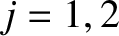indexes the columns. Furthermore,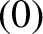denotes a null vector, and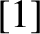denotes an identity matrix.

Equations (2.175) and (2.176) can be written in the compact form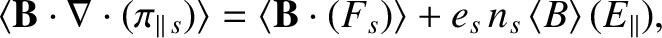(2.177)

where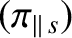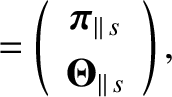(2.178)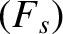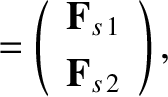(2.179)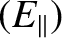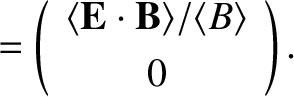(2.180)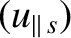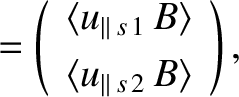(2.181)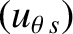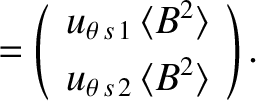(2.182)

Equations (2.170)–(2.174), (2.181), and (2.182) can be combined to give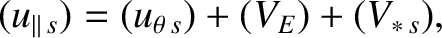(2.183)

where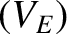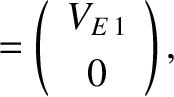(2.184)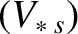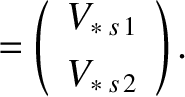(2.185)

It is also helpful to define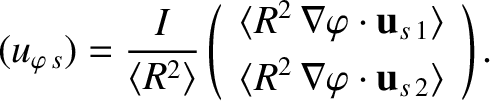(2.186)

It follows from Equations (2.130), (2.134)–(2.137), (2.165), (2.166), and (2.171)–(2.174) that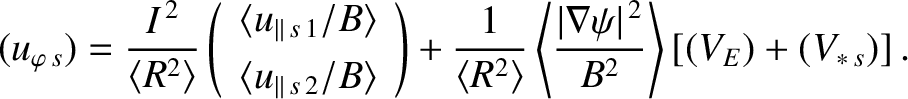(2.187)

However, Equations (2.170) and (2.182) yield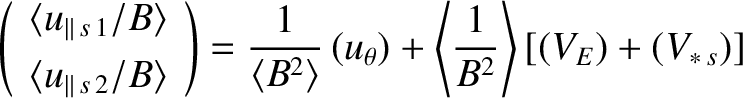(2.188)

Finally, the previous two equations can be combined to give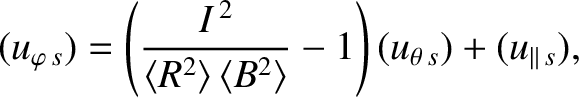(2.189)

where use has been made of Equations (2.130) and (2.183).